8th Grade Math Home > Teacher Resources > Activities > View Tubes: Teacher Notes

View Tubes: Teacher Notes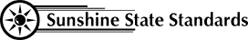MA.8.S.3.1
MA.912.A.3.11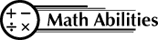Conceptual Understanding

Data Collection
Graphing
Line of Best Fit
Interpret Data

Procedural Knowledge

Organize Data
Graph Data
Make Predictions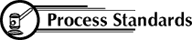Problem Solving
Reasoning
Connections
Representation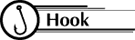Does the length of a viewing tube, its diameter, or the distance from an object affect the type of data collected and the resulting graph?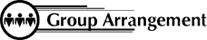Work in groups of four or five.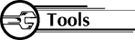Each group needs:

• Measuring Tape placed vertically on the wall from the floor upward.
• Assorted tubes with varying lengths and diameters to correspond to the investigation assigned to each group
• Tape to mark standing positions on the floor in front of the measuring tape
• Grid paper for each group1. Students will work in groups at designated "viewing stations."
2. Each viewing station has a measuring tape on the wall and standing positions marked on the floor in front of the wall.
3. Each student standing at indicated distances from the wall will use a tube to view the measuring tape and then describe to other group members what portion of the poster is visible.
4. Other group members will measure the height of the described viewable portion to the nearest inch.
5. Data is recorded as collected for each member of the group.
6. Graphs of best-fit lines or curves are drawn and interpretations made.
7. Investigating the relationship between the dimensions of the tubes, slopes, and intercepts should reveal:
1. When tube length and diameter are constant, the viewable height is a linear function of the distance from the wall. The intercept is the diameter of the tube.
2. When distance from the wall and tube length are constant, the viewable height is a linear function of the diameter of the tube.
3. When the distance from the wall and tube diameter are constant, the viewable height is a nonlinear function of the length of the tube.

Investigation A

1. Collect the Data: Each member of the group will view the poster with the given tubes at varying distances from the wall. Others will mark the top and bottom of the described portion to determine the measurement in inches of the viewing height. Calculate the average visible height for each of the distances.
2. Graph the Data: Choose appropriate labels and scales for the horizontal and vertical axes. Plot the data as ordered pairs, (x, y).
3. Read the Results: Looking at your points, do they seem to lie along a line or a curve? Draw the line that best fits your data.
4. Describe in words how to determine the height of the visible portion if you know the distance from the wall.
5. Describe by equation how to determine the height of the visible portion (y) if you know the distance from the wall (x).
y =
6. Predict the height of the visible portion, if you were standing 10 feet
from the wall.
7. Predict the distance from the wall you would have to stand in order to see a 25-inch portion of the poster.

Viewing Height:
Same Tube Length & Tube Diameter
Varying Distances from Wall

 Student name Distance from Poster 1 foot 2 feet 3 feet 4 feet 5 feet 6 feet 7 feet 8 feet Total

Investigation B
1. Collect the Data: Each member of the group will view the poster with the given tubes at varying distances from the wall. Others will mark the top and bottom of the described portion to determine the measurement in inches of the viewing height. Calculate the average visible height for each of the distances.
2. Graph the Data: Choose appropriate labels and scales for the horizontal and vertical axes. Plot the data as ordered pairs, (x, y).
3. Read the Results: Looking at your points, do they seem to lie along a line or a curve? Draw the line that best fits your data.
4. Describe in words how to determine the height of the visible portion if you know the diameter of the tube.
5. Describe by equation how to determine the height of the visible portion (y) if you know the diameter of the tube (x).
y =
6. Predict the height of the visible portion for a tube with a diameter of five inches.
7. Predict the diameter of a tube that would allow you to see a 10-inch portion of the poster.

Viewing Height:
Same Distance from Wall & Tube Length
Varying Diameters

 Student Name Diameter of Tube 1" 2" 3" 4" 5" 6" Average

Investigation C
1. Collect the Data: Each member of the group will view the poster with the given tubes varying in length, a designated distance from the wall. Others will mark the top and bottom of the described portion to determine the measurement in inches of the viewing height. Calculate the average visible height for each of the lengths.
2. Graph the Data: Choose appropriate labels and scales for the horizontal and vertical axes. Plot the data as ordered pairs, (x, y).
3. Read the Results: Looking at your points, as the length of the tube increases, what is happening to the height of the visible portion of the picture?
4. Would the height of the portion ever decrease to zero? Why?
5. What height does the line seem to be approaching as a minimum?

Viewing Height
Same Tube Diameter & Distance from Wall
Varying Length

 Student Name Length of Tube 4 inches 7 inches 22 inches 33 inches Average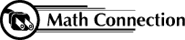As a result of this activity, students learn to analyze data that they have collected, look for relationships, and make predictions.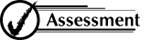Have students answer the following questions:

1. Explain how you knew the graphs were or were not linear.
2. Predict the height of the visible portion of the measuring tape if you were standing 15 feet from the wall.
3. Predict the distance you would have to stand from the wall in order to see a 30-inch portion of the measuring tape.
4. Predict the height of the visible portion for the tube with a diameter of 7.5 inches.
5. Predict the diameter of a tube that would allow you to see a 20-inch portion of the measuring tape.

 8th Grade Math Home > Teacher Resources > Activities > View Tubes: Teacher Notes